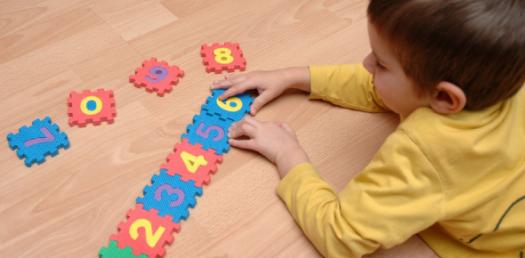# Play With Mathematics: Quantitative And Reasoning Quiz!

10 Questions | Attempts: 1382SettingsAre you smart enough to solve the mathematical reasoning and quantitative questions in mind without using a calculator? If yes, then do attempt this quiz to prove your worth by taking this challenge. There is a list of 10 difficult questions which you have to solve without using any calculator or reference. So, let's try out the quiz. All the best!

• 1.
Which of the following groups contains equivalent values expressed in the various forms?
• A.

0.75, 70%, 3/4

• B.

0.15, 15%, 3/20

• C.

0.166, 16%, 1/6

• D.

0.50, 50%, 1/5

• 2.
A car drove 3.5 hours and travelled a total of 242 miles. How fast was the car going, how many MPH(miles per hour) was the car going?
• A.

63 MPH

• B.

65 MPH

• C.

67 MPH

• D.

69 MPH

• 3.
Solve the expression below. 4*2 + 3(6-2) + 81
• A.

100

• B.

101

• C.

104

• D.

96

• 4.
X = 10 apples y = 5 oranges Using the data below, figure out how many oranges and apples were picked by Kevin and Jenny combined. Kevin picked x x x x x apples and y y y y oranges. Jenny picked x x x apples and y oranges.
• A.

80 apples and 25 oranges

• B.

50 apples and 20 oranges

• C.

30 apples and 5 oranges

• D.

None of the above.

• 5.
The Least Common Multiple of two numbers is the smallest number (not including zero) that is a multiple of both. What this the LCM of 3 and 4?
• A.

0

• B.

12

• C.

21

• D.

24

• 6.
The Greatest Common factor is the greatest factor that divides two numbers. What is the greatest common factor of 18 and 36?
• A.

2

• B.

6

• C.

9

• D.

18

• 7.
Kevin walks home from school and it takes him an average of 1/2 hour per mile. What is a reasonable amount of time for him to walk to the movies which are 6.0 miles away?
• A.

2 hours

• B.

3 hours

• C.

3.5 hours

• D.

4.0 hours

• 8.
There are 9 classes of students with 37 students in each class along with 1 teacher for each class. Which of the following operations would you do to find out how many students and teachers were in the gym?
• A.

(9*37) + 1

• B.

(9*37) + 37

• C.

(9*37) + 9(1)

• D.

(9*9) + 37

• 9.
Order the following four numbers in order from greatest to smallest. 1/2 0.55 56% 0.15
• A.

1/2> 0.55> 56%> 0.15

• B.

0.15< 1/2< 0.55< 56%

• C.

56%> 0.55> 1/2> 0.15

• D.

None of the above.

• 10.
John is making 40 pies and he needs 1.5 cups of flour for each pie. If he buys a bags of flour with 10 cups of flour in each bag. How many bags total will he need to make the 40 pies?
• A.

4 bags

• B.

5 bags

• C.

6 bags

• D.

7 bags

## Related TopicsBack to top
×

Wait!
Here's an interesting quiz for you.